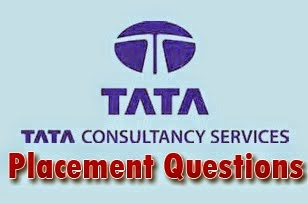Breaking News
Home / Placement Papers / TCS / TCS Sample Aptitude Questions

# TCS Sample Aptitude Questions

## TCS Sample Aptitude Questions And Answers1.In a 200 meters race, A beats B by 20 meters, while in a 100 meters race, B beats C by 5 meters. A beats C in a kilometer race by

1.105 meters
2.225 meters
3.205 meters
4.145 meters

When A = 200, B = 180.
When B = 100, C = 95.
Make B = 900. A will be 1000. C will be 855.

2.A person purchases tomatoes from each of the 4 places at the rate of 1 kg, 2 kg, 3 kg, 4 kg per rupee respectively. On an average he has purchased x kg of tomatoes per rupee. Then the value of x is

1.2
2.2.5
3.1.92
4.None of these

x = 10/4 = 2.5

3.The value of 51/4 × (125)0.25 is

1.5
2.25
3.50
4.10

51/4 × (125)0.25 =51/4 + 3/4 =51 =5

4.A sum of money doubles itself in 7 years. In how many years it becomes four fold?

1.35 years
2.28 years
3.14 years
4.21 years

Double in seven years.
So 4 times will be in = 7 × (4 − 1) = 21 years.

5.A fast train takes 3 hours less than a slow train for a journey of 600 km, If the speed of the slow train is 10 km/hr less than that of the fast train, the speeds of the two trains are

1.60 km/hr and 70 km/hr
2.50 km/hr and 60 km/hr
3.40 km/hr and 50 km/hr
4.30 km/hr and 40 km/hr

(600/x)-(600/x+10)=(3/1)
Solving this, we get x = 40

6.When 75% of a number is added to 75, the result is the same number. The number is

1.150
2.300
3.100
4.450

Let the number be equal to x.
3x/4 +75 = x
Hence x=300

7.If 5 spiders can catch five flies in five minutes, how many flies can hundred spiders catch in 100 minutes?

1.100
2.1000
3.500
4.2000

5 × 5 × x = 100 × 100 × 5 ⇒ x = 2000.

8.A car travels 25km an hour faster than a bus for a journey of 500km. If the bus takes 10 hours more than the car, then the speeds of the bus and the car are

1.25km/hr and 40km/hr respectively
2.25km/hr and 60 km/hr respectively
3.25km/hr and 50km/hr respectively
4.None of these

Let the speeds of bus and car be x and y. Here, y = x +25
As per the question, (500/x)-(500/x+25)=10
⇒ 500(x + 25) − 500(x) =10x(x+25) ⇒ x = 25, y = 50.
For such questions, you can also use direct substitution from the given choices

9.An article is bought for Rs. 600 and sold for Rs. 750. The gain percent is:

1.20%
2.25%
3.30%
4.None10.If P : Q = 3 : 4 and Q : R = 5 : 6, then P : Q : R is:

1.3 : 4 : 6
2.3 : 5 : 6
3.15 : 20 : 24
4.5 : 6 : 3